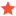VB.NET Setting pose UR10e [FIXED]awileyJunior MemberPosts: 1 Threads: 1 Joined: Apr 2019 Reputation: 2 04-23-2019, 06:21 PM (This post was last modified: 04-23-2019, 08:38 PM by awiley.) When I try and set a pose for the UR10e it does not move to the correct rpw. "Dim testPoint As Mat = Mat.transl(x, y, z) * Mat.rotx(Deg_to_Rad(i)) * Mat.roty(Deg_to_Rad(j)) * Mat.rotz(Deg_to_Rad(k))"  is the Matrix that I am trying to set the pose to. It works if one of the numbers is different than 0, but not if multiple are different than 0. Thank you for any help! Fix: Private Function Deg_to_Rad(deg As Double) As Double     Return ((deg * Math.PI) / 180) End Function Dim moveTo As Double() = {x, y, z, Deg_to_Rad(i), Deg_to_Rad(j), Deg_to_Rad(k)} Dim testPoint As Mat = Mat.URToPose(moveTo) ROBOT.setPoseFrame(FRAME) ROBOT.setPose(testPoint) This will allow the robot to move to a point and then set a target, this will let you set the XYZ and RPW. Find ReplyJeremyModeratorPosts: 1,800 Threads: 2 Joined: Oct 2018 Reputation: 69 04-25-2019, 05:41 AM Pretty good job. Up voted! Jeremy Find Reply